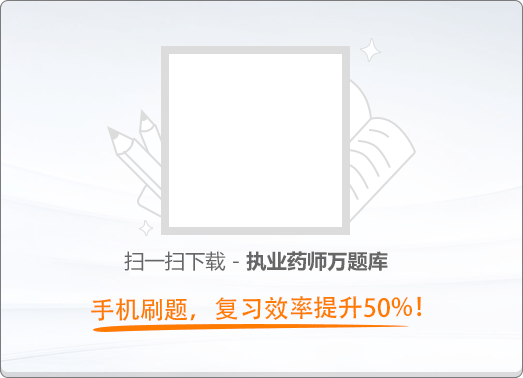90:00《药事管理与法规》历年真题试卷（二）

1
(单项选择题)

• A.

公共卫生体系

• B.

医疗服务体系

• C.

医疗保障体系

• D.

药品供应保障体系

• E.

医药卫生监管体系

• A
• B
• C
• D
• E

3
(单项选择题)

• A.

对特定疾病有特殊疗效的

• B.

对特定疾病有显著疗效的

• C.

用于预防特殊疾病的

• D.

用于治疗特殊疾病的

• E.

已申请专利的中药品种

• A
• B
• C
• D
• E

6
(单项选择题)

• A.

开办药品生产企业，应当经国家药品监督管理部门批准并发给《药品生产许可证》

• B.

经县级以上药品监督管理部门批准，药品生产企业可以接受委托生产药品

• C.

药品生产企业改变影响药品质量的生产工艺的，必须报原批准部门审核批准

• D.

经具有合法资格的药品生产企业之间协商一致，可以委托生产药品

• E.

采用企业内定的中药饮片炮制规范炮制中药饮片

• A
• B
• C
• D
• E

7
(单项选择题)

• A.

具有依法经过资格认定的药学技术人员

• B.

具有与所经营药品相适应的营业场所、设备、仓储设施、卫生环境

• C.

具有与所经营药品相适应的质量管理机构或者人员

• D.

具有保证所经营药品质量的规章制度

• E.

具有能对所经营药品进行质量检验的人员以及必要的仪器

• A
• B
• C
• D
• E

8
(单项选择题)

• A.

生物制品

• B.

麻醉药品

• C.

精神药品

• D.

医疗用毒性药品

• E.

放射性药品

• A
• B
• C
• D
• E

9
(单项选择题)

• A.

变质的药品

• B.

被污染的药品

• C.

所标明的适应症或者功能主治超出规定范围的药品

• D.

未注明生产批号的药品

• E.

所含成分与国家药品标准规定的成分不符的药品

• A
• B
• C
• D
• E

10
(单项选择题)

• A.

药物研究所的药品检验人员

• B.

药品检验机构

• C.

药品监督管理部门

• D.

药品检验机构的工作人员

• E.

药品监督管理部门的公务员

• A
• B
• C
• D
• E

11
(单项选择题)

• A.

将经依法批准制备的制剂调配给本院门诊患者使用

• B.

在本院病房走廊张贴客观宣传该制剂疗效的广告

• C.

依法取得《医疗机构制剂许可证》，经所在地省级卫生行政部门同意后，即开始配制本院临床需用的制剂

• D.

因突发疫情，应乙医院请求，将经依法批准制备的制剂调剂给乙医院使用，事后及时向省级药品监督管理部门报告

• E.

因乙医院抢救患者急需，而市场没有供应，将经依法批准制备的制剂调剂给乙医院使用，同时向省级卫生行政部门报告

• A
• B
• C
• D
• E

13
(单项选择题)

• A.

取得《药品经营许可证》之日起7个工作日

• B.

取得《药品经营许可证》之日起15个工作日

• C.

取得《药品经营许可证》之日起30日内

• D.

取得《药品经营许可证》之日起3个月内

• E.

取得《药品经营许可证》之日起6个月内

• A
• B
• C
• D
• E

14
(单项选择题)

《麻醉药品、精神药品管理条例》关于麻醉药品监督管理，说法正确的是（　）。

• A.

麻醉药品目录由公安部、卫生部制定、调整并公布

• B.

麻醉药品目录由国家食品药品监督管理总局制定、调整并公布

• C.

麻醉药品目录由国家食品药品监督管理总局会同公安部、卫生部制定、调整并公布

• D.

麻醉药品药用原植物由国家食品药品监督管理总局监督管理

• E.

麻醉药品流入非法渠道的行为由国家食品药品监督管理总局查处

• A
• B
• C
• D
• E

15
(单项选择题)

• A.

全国性批发企业可以经营麻醉药品的原料药

• B.

全国性批发企业可以经营第一类精神药品的原料药

• C.

全国性批发企业和区域性批发企业都可经营第二类精神药品

• D.

区域性批发企业可以直接从定点生产企业购进麻醉药品

• E.

区城性批发企业可以经国家食品药品监督管理总局批准跨省销售麻醉药品

• A
• B
• C
• D
• E

16
(单项选择题)

• A.

省级卫生行政部门

• B.

设区的市级卫生行政部门

• C.

省级药品监督管理部门

• D.

设区的市级药品监督管理部门

• E.

卫生行政部门会同药品监督管理部门

• A
• B
• C
• D
• E

17
(单项选择题)

• A.

毒性药品的年度生产计划由国家药品监督管理部门批准

• B.

生产企业应按批准的生产计划生产

• C.

由医药专业人员负责生产、配制和质量检验

• D.

每次配料必须2人以上复核

• E.

生产原料和成品数每次记录，经手人需签字备查

• A
• B
• C
• D
• E

18
(单项选择题)

• A.

省级疾病预防控制机构通知县级疾病预防控制机构立即停止接种、分发该疫苗

• B.

县级疾病预防控制机构接到通知后立即停止接种、分发该疫苗

• C.

县级疾病预防控制机构立即向县级卫生行政部门和药品监督管理部门报告

• D.

县级疾病预防控制机构应疫苗生产企业要求，将该批疫苗退回生产企业查明质量问题

• E.

接到报告的药品监督管理部门对该批疫苗依法采取查封，扣押等措施

• A
• B
• C
• D
• E

19
(单项选择题)

• A.

2年，3个月

• B.

3年，3个月

• C.

3年，6个月

• D.

5年，1个月

• E.

5年，6个月

• A
• B
• C
• D
• E

20
(单项选择题)

• A.

全国零售指导价销售

• B.

零差率销售

• C.

在进价的基础上加价5%销售

• D.

在进价的基础上加价10%销售

• E.

在进价的基础上加价15%销售

• A
• B
• C
• D
• E

21
(单项选择题)

• A.

临床常用、价格合理、中西医并重、基本保障、市场供应充足

• B.

临床必需、安全有效、价格合理、使用方便、市场能够保证供应

• C.

保证品种和质量、引入竞争机制、合理控制成本、方便购药和便于管理

• D.

防治必需、安全有效、质诚优先、价格低廉、中西医并重、临床常用和基本能够配备

• E.

防治必需、安全有效、价格合理、使用方便、中西药并重、基本保障、临床首选和基层能够配备

• A
• B
• C
• D
• E

22
(单项选择题)

• A.

医疗机构不能推荐使用非处方药

• B.

处方药经审批可以在大众媒体上进行广告宣传

• C.

非处方药说明书由省级药品监督管理部门批准

• D.

每个销售基本单元包装必须附有标签和说明书

• E.

根据药品的给药途径不同，非处方药分为甲乙两类

• A
• B
• C
• D
• E

23
(单项选择题)

• A.

红色专有标识用于甲类非处方药药品

• B.

绿色专有标识用于乙类非处方药药品

• C.

红色专有标识用于药品批发企业的指南性标志

• D.

绿色专有标识用于经营非处方药零售企业的指南性标识

• E.

非处方药说明书上单色印刷非处方药专有标识，并在其下标示“甲类”或“乙类”字样

• A
• B
• C
• D
• E

25
(单项选择题)

• A.

新药监测期内的药品

• B.

经批准上市5年内的新药

• C.

首次进口5年内的药品

• D.

国家基本药物目录中的药品

• E.

省级以上药品监督管理部门要求的特定药品

• A
• B
• C
• D
• E

27
(单项选择题)

• A.

药品生产企业

• B.

药品经营企业

• C.

医疗机构

• D.

药品检验机构

• E.

药物研究机构

• A
• B
• C
• D
• E

28
(单项选择题)

• A.

企业法定代表人或企业负责人

• B.

质量管理部门负责人

• C.

质量管理人员

• D.

质量验收人员

• E.

负责拆零销售人员

• A
• B
• C
• D
• E

31
(单项选择题)

• A.

药品生产、经营企业应对其药品购销行为负责

• B.

药品生产、经营企业可派出销售人员以本企业名义从事药品购销活动

• C.

药品生产企业可以销售本企业生产的药品

• D.

药品生产企业可以销售本企业经许可受委托生产的药品

• E.

药品生产、经营企业对其销售人员以本企业名义从事的药品购销行为承担法律责任

• A
• B
• C
• D
• E

32
(单项选择题)

• A.

提供互联网交易服务的企业必须严格审核参与互联网交易的药品的合法性

• B.

向个人消费者提供互联网交易服务的企业可以在网上销售本企业经营的全部药品

• C.

通过自身网站与本企业成员之外的其他企业进行互联网交易的药品生产企业只能交易本企业生产的药品

• D.

通过自身网站进行互联网药品交易服务的药品经营企业不得利用自身网站提供其他互联网交易服务

• E.

提供互联网药品交易服务的企业必须审核交易各方的资格证明文件并备案

• A
• B
• C
• D
• E

33
(单项选择题)

• A.

按非限制使用级管理

• B.

按限制使用级管理

• C.

按特殊使用级管理

• D.

禁止列入医疗机构供应目录

• E.

禁止列入省级抗菌药物分级管理目录

• A
• B
• C
• D
• E

34
(单项选择题)

• A.

1年内不得受理其申请，并处1万元以上3万元以下罚款

• B.

2年内不得受理其申请，并处1万元以上3万元以下罚款

• C.

3年内不得受理其申请，并处1万元以上3万元以下罚款

• D.

4年内不得受理其申请，并处1万元以上3万元以下罚款

• E.

5年内不得受理其申请，并处1万元以上3万元以下罚款

• A
• B
• C
• D
• E

35
(单项选择题)

• A.

中专以上药学或者相关专业学历

• B.

大专以上药学或者相关专业学历

• C.

本科以上药学或者相关专业学历

• D.

大专以上药学学历

• E.

本科以上药学专业学历

• A
• B
• C
• D
• E

36
(单项选择题)

• A.

法人变更

• B.

医疗机构类别变更

• C.

机构注册地址变更

• D.

制剂配制地址变更

• E.

医疗机构名称变更

• A
• B
• C
• D
• E

37
(单项选择题)

• A.

药品说明书应当列出全部活性成分或者组方中的全部中药药味

• B.

药品说明书禁止使用未经注册的商标

• C.

注射剂的说明书应当列出所用的全部辅料名称

• D.

口服缓释制剂的说明书应当列出所用的全部辅料名称

• E.

非处方药说明书应当列出所用的全部辅料名称

• A
• B
• C
• D
• E

40
(单项选择题)

• A.

药店不知道该产品为假名牌，不应承担责任

• B.

药店不是假名牌的生产者，不应承担责任

• C.

该产品未经药品监督管理部门认定和检验，药店不应承担责任

• D.

王某对该产品有怀疑仍接受了服务，应承担部分责任

• E.

药店违反了保证商品和服务安全的义务，应当承担责任

• A
• B
• C
• D
• E